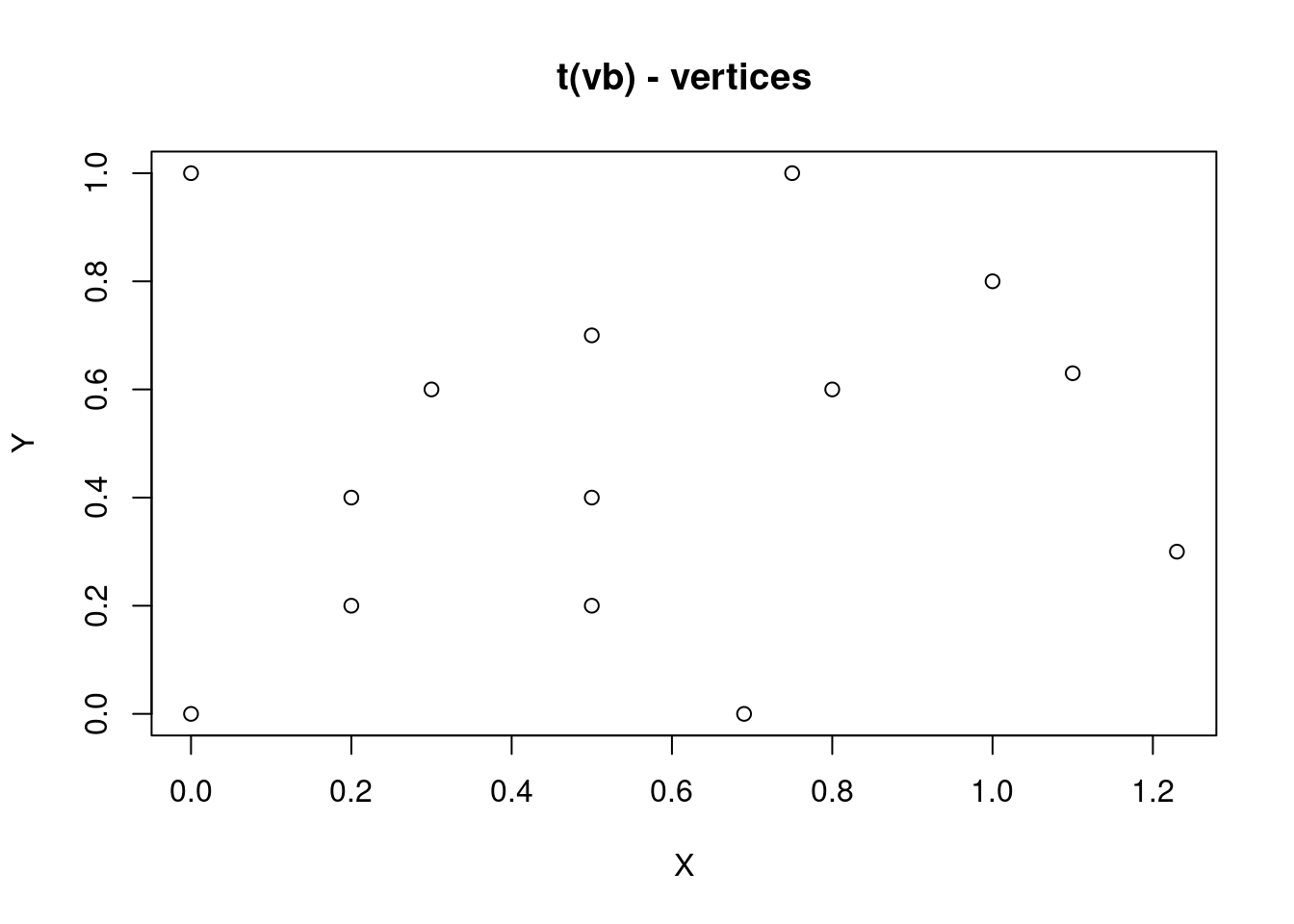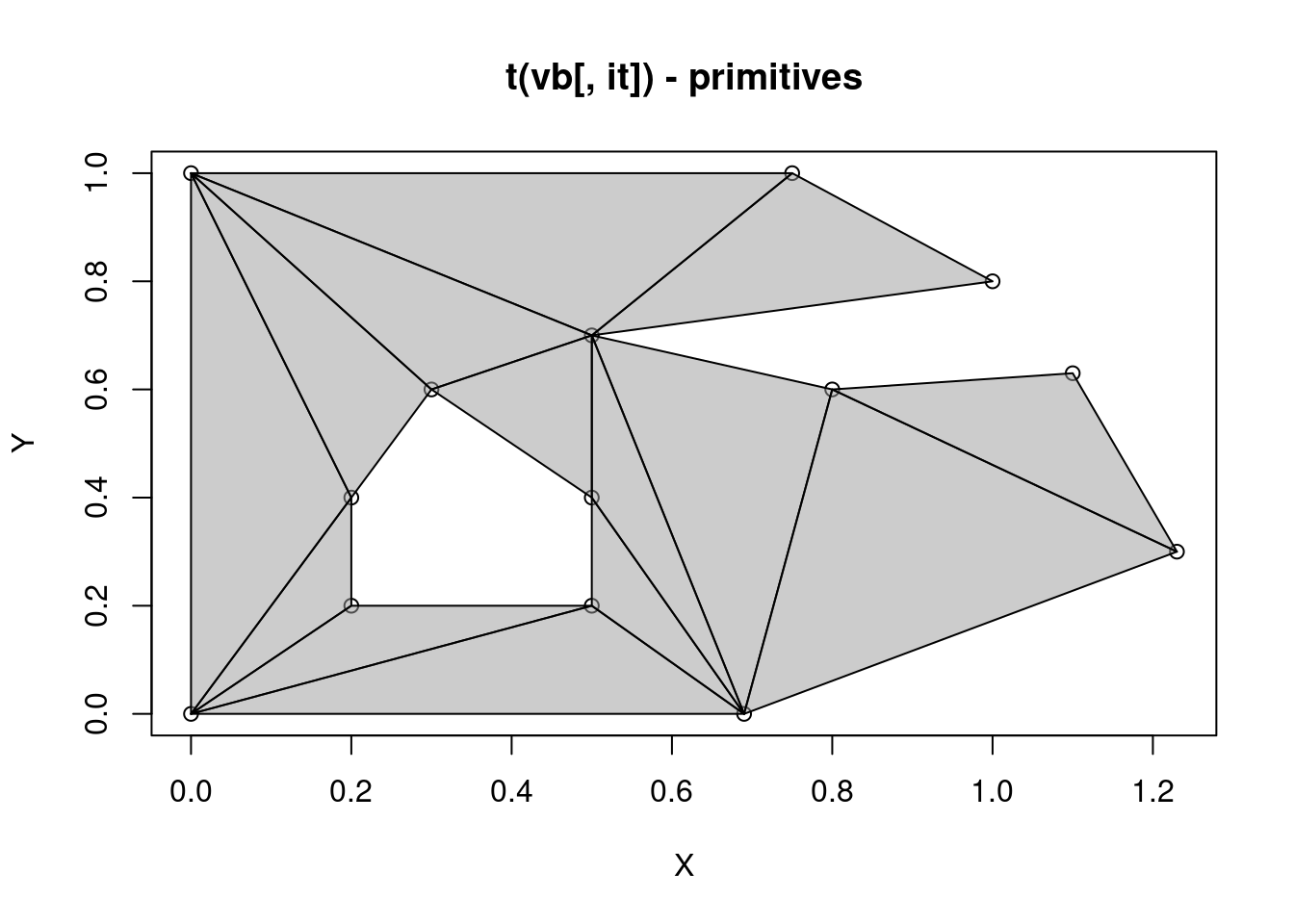# mesh3d - recent changes in rgl workhorse format

news
code
Author

Michael D. Sumner

Published

2019-05-29

PLEASE NOTE (April 2022): this post has been migrated from an old site, and some details may have changed. There might an update to this post to reflect the rgl package as it is now. —

This post describes the mesh3d format used in the rgl package and particularly how colour properties are stored and used. There are recent changes to this behaviour (see ‘meshColor’), and previously the situation was not clearly documented.

## rgl

The rgl package has long provided interactive 3D graphics for R. The neat thing for me about 3D graphics is the requirement for mesh forms of data, and the fact that meshes are extremely useful for very many tasks. When we plot data in 3D we necessarily have to convert the usual spatial types into mesh forms. You can see me discuss that in more detail in this talk.

## The mesh3d format

Here is an example of a mesh3d object, it stores two polygonal areas in a form ready for 3D graphics.

``````mesh0 <- structure(list(vb = structure(c(0, 0, 0, 1, 0, 1, 0, 1, 0.75,
1, 0, 1, 1, 0.8, 0, 1, 0.5, 0.7, 0, 1, 0.8, 0.6, 0, 1, 0.69,
0, 0, 1, 0.2, 0.2, 0, 1, 0.5, 0.2, 0, 1, 0.5, 0.4, 0, 1, 0.3,
0.6, 0, 1, 0.2, 0.4, 0, 1, 1.1, 0.63, 0, 1, 1.23, 0.3, 0, 1), .Dim = c(4L, 14L)),
it = structure(c(1L, 8L, 12L, 9L, 8L, 1L, 7L, 6L, 5L,
5L, 4L, 3L, 2L, 1L, 12L, 9L, 1L, 7L, 5L, 3L, 2L, 2L, 12L, 11L,
10L, 9L, 7L, 5L, 2L, 11L, 10L, 7L, 5L, 5L, 11L, 10L, 6L, 7L,
14L, 14L, 13L, 6L), .Dim = c(3L, 14L)),
primitivetype = "triangle",
material = list(),
normals = NULL,
texcoords = NULL),
class = c("mesh3d", "shape3d"))

str(mesh0)``````
``````List of 6
\$ vb           : num [1:4, 1:14] 0 0 0 1 0 1 0 1 0.75 1 ...
\$ it           : int [1:3, 1:14] 1 8 12 9 8 1 7 6 5 5 ...
\$ primitivetype: chr "triangle"
\$ material     : list()
\$ normals      : NULL
\$ texcoords    : NULL
- attr(*, "class")= chr [1:2] "mesh3d" "shape3d"``````

(It’s not obvious about the polygons, please bear with me).

The following characterizes the structure.

• two matrix arrays `vb` and `it`
• `vb` has 4 rows and 14 columns, and contains floating point numbers
• `it` has 3 rows and 14 columns, and contains integers (starting at 1)
• a `primitivetype` which is “triangle”
• an empty list of `material` propertes (this is the missing link for the polygons)
• a `NULL` value for `normals` and `texcoords`, these won’t be discussed further (but see `?quadmesh::quadmesh` for texture coordinates from spatial)
• a class, this object is a `mesh3d` and inherits from `shape3d`

The `vb` array is the vertices, these are the corner coordinates of the elements of the mesh.

``plot(t(mesh0\$vb), main = "t(vb) - vertices", xlab = "X", ylab = "Y")``The elements of this mesh are triangles, and these are specified by the index array `it`. Elements of a mesh are called primitives, hence the `primitivetype` here.

``````plot(t(mesh0\$vb), main = "t(vb[, it]) - primitives", xlab = "X", ylab = "Y")
polygon(t(mesh0\$vb[, rbind(mesh0\$it, NA)]), col = rgb(0.6, 0.6, 0.6, 0.5))``````## Transpose

These matrix arrays are transpose the way we usually use them in R, for now just remember that you must `t()`ranspose them for normal plotting, e.g. `plot(t(mesh0\$vb[1:2, ]))` will give the expected scatter plot of the vertices. The reason these arrays are transpose is because each coordinate value is then contiguous in memory, each Y value is right next to its counterpart X, and Z (and W), and `vb[it, ]` provides a flat vector of XYZW values in a continuous block - this is a very important efficiency, and help explains why computer graphics use elements in a mesh form like this.

## Colours

Unsurprisingly, if we set the `material` property to a constant we get a constant colour.

``````widgetfun <- function() {
view3d(0, phi = 8)
rglwidget()
}
mesh0\$material\$color <- "red"
library(rgl)
clear3d()
shade3d(mesh0, lit = FALSE);
widgetfun()``````

In the usual R way our singleton colour value is magically recycled across every part of the shape, and it’s all red. But, is it recycled by vertices or by primitive? Until recently it was only possible to tell by trying (or reading the source code).

Here I think it’s easy to see that the two colours are specified at the vertices, and they bleed across each triangle accordingly. We also get a warning that the behaviour has recently changed.

``````clear3d()
mesh0\$material\$color <- c("firebrick", "black")
material3d(lit = FALSE)
widgetfun()``````

The default is to `meshColor = "vertices"`, so let’s specify `faces`.

``````clear3d()
mesh0\$material\$color <- c("firebrick", "dodgerblue")
material3d(lit = FALSE)
shade3d(mesh0, meshColor = "faces")
widgetfun()``````

Sometimes we get neighbouring triangles with the same colour, so let’s also add the edges.

``````mesh0\$vb[3, ] <- 0.01  ## vertical bias avoids z-fighting
## material properties here override the recycling of internal colours
## onto edges
wire3d(mesh0, lwd = 5, color = "black")
widgetfun()``````

If we go a bit further we can see the original arrangement for this shape, two individual polygons that share a single edge.

This only works because I happen to know how this was created, and I know how this control of behaviour occurs in new rgl.

There are 12 triangles in the first polygon, and 2 in the second. (The original polygons can be seen here (left panel)).

``````clear3d()
mesh0\$material\$color <- rep(c("firebrick", "dodgerblue"), c(12, 2))
shade3d(mesh0, meshColor = "faces", lit = FALSE)
widgetfun()``````

If we treat the colours as applying to each vertex, then we needed to propagate it to each vertex around each face (triangle), and this is what rgl now calls legacy behaviour.

``````clear3d()
mesh0\$material\$color <- rep(rep(c("firebrick", "dodgerblue"), c(12, 2)), each = 3)
shade3d(mesh0, meshColor = "legacy", lit = FALSE)
widgetfun()``````

We cannot recreate this effect with `meshColor = "vertices"`, because each of our vertices is actually unique. (It could be done by making the vb array every repeated vertex, and updating the index array but I can’t summon this up atm).

``````clear3d()
mesh0\$material\$color <- rep_len(c("firebrick", "dodgerblue"), length.out = ncol(mesh0\$vb))
shade3d(mesh0, meshColor = "vertices", lit = FALSE)
widgetfun()``````

## Primitives

The other kind of element supported by mesh3d is a quad, specified by an `ib` array with 4 rows (`ib` versus `it`, 4 vertices versus 3) and the `primitivetype = "quad"`.

The `it` values are an index into, i.e. the column number of the vertex array. The vertices, or coordinates, are stored by column in this structure, whereas normally we would store a coordinate per row.

When I first explored mesh3d I was looking at a quad type mesh - and I was completely confused. Both `vb` and `ib` had four rows, and so while I understood that a quad must have 4 vertices (4 index values for every primitive), I did not understand why the vertices also had four rows.

(There are other kinds of primitives in common use are edge, point, tetrahedron - but rgl has no formal class for these - in practice the edge type is referred to as segment in rgl, and tetrahedra are approximated by enclosing their shape with triangles).

## Why does the vertex array have 4 rows?

All mesh3d objects have a `vb` array, and it always includes 4 rows.

The reason there are 4 rows in the vertex array is that these are homogeneous coordinates which …

are ubiquitous in computer graphics because they allow common vector operations such as translation, rotation, scaling and perspective projection to be represented as a matrix by which the vector is multiplied

… yeah. For our purposes just think

X, Y, Z in the usual sense and set `W = 1`.

(Do not set `W = 0` because your data will vanish to infinity when plotted with rgl, which is what those math folks are saying more or less).

Now let’s get a quad type mesh from the real world.

``````## remotes::install_github("hypertidy/ceramic")
library(ceramic)
topo <- cc_elevation(raster::extent(-72, -69, -34, -32), zoom = 6)``````
``````Preparing to download: 1 tiles at zoom = 6 from
https://api.mapbox.com/v4/mapbox.terrain-rgb/``````
``````qm <- quadmesh::quadmesh(topo)

str(qm)``````
``````List of 8
\$ vb             : num [1:4, 1:60225] -8015493 -3761925 0 1 -8014270 ...
..- attr(*, "dimnames")=List of 2
.. ..\$ : chr [1:4] "x" "y" "z" "1"
.. ..\$ : NULL
\$ ib             : int [1:4, 1:59732] 1 2 277 276 2 3 278 277 3 4 ...
\$ primitivetype  : chr "quad"
\$ material       : list()
\$ normals        : NULL
\$ texcoords      : NULL
\$ raster_metadata:List of 7
..\$ xmn  : num -8015493
..\$ xmx  : num -7680393
..\$ ymn  : num -4028537
..\$ ymx  : num -3761925
..\$ ncols: int 274
..\$ nrows: int 218
..\$ crs  : chr "+proj=merc +lon_0=0 +k=1 +x_0=0 +y_0=0 +R=6378137 +units=m +no_defs"
\$ crs            : chr "+proj=merc +lon_0=0 +k=1 +x_0=0 +y_0=0 +R=6378137 +units=m +no_defs"
- attr(*, "class")= chr [1:3] "quadmesh" "mesh3d" "shape3d"``````

This topographic raster from near Santiago is now a mesh3d subclassed to quadmesh. This adds two properties `raster_metadata` and `crs`, which under limited conditions allows reconstruction of the original raster data. To drop back to a generic mesh3d the easiest is to reproject the data.

``````##remotes::install_github("hypertidy/reproj")
library(reproj)
qm_ll <- reproj(qm, "+proj=longlat +datum=WGS84")``````
``````Warning in reproj.quadmesh(qm, "+proj=longlat +datum=WGS84"): quadmesh raster
information cannot be preserved after reprojection, dropping to mesh3d class``````

This is a lossless reprojection, as it is equivalent to `sf::sf_project(t(qm\$vb[1:2, ]), from = qm\$crs, to = "+proj=longlat +datum=WGS84")` or with `rgdal::project(, qm\$crs, inv = TRUE)`.

We can plot this in the usual way with rgl, or see upcoming features in the mapdeck package.

``````clear3d()
shade3d(qm_ll, lit = TRUE, col = "grey")
aspect3d(1, 1, 0.1);
view3d(0, phi = -60)
rglwidget()``````